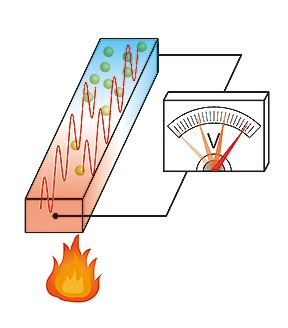Can we convert temperature differences into electricity? - School of Science, the University of Tokyo
Nov 15, 2018

## Can we convert temperature differences into electricity?

#### (Professor, Department of Physics)

This summer is extremely hot! (This article was written in July 2018.) Summer in Japan may continue to be this hot from now on, and there will soon come a time when we can generate electricity at every house by using the temperature difference between the extremely hot air outside and the air-conditioned air inside rooms. A considerable amount of usable heat is wasted even today, such as exhaust heat from underground trains and blast furnaces. Although the realization of effective usage of these temperature differences is an engineering issue, the scientific problem is to identify what kind of mechanism can convert temperature differences into electric voltage. This is generally known as a thermoelectric problem.

As illustrated in the figure below, the thermoelectric phenomenon in which temperature differences are converted into voltage is one of the fundamental problems in physics; however, alongside recent remarkable developments in experimental research, various problems that need to be resolved theoretically have also emerged. In order to explain further, I will begin with the linear response theory in condensed matter physics.

One of the main research areas in physics concerns the investigation of states of matter, such as elucidating phase diagrams that illustrate how variations in pressure or temperature affect states of matter, and determining whether or not substances will become superconductors. These are problems in thermal equilibrium.

The advanced problem lies in the non-equilibrium state. However, if the degree of non-equilibrium is too large, current statistical mechanics or thermodynamics cannot be applied. One of the fundamental difficulties is that the temperature itself is undefinable in the non-equilibrium state. A first step to approaching the non-equilibrium problems is to think about a situation in which there is only a slight deviation from the equilibrium. This is, in other words, a method to investigate how a system responds when a small perturbation is applied to the equilibrium state. This method is called the linear response theory and was conceptualized in 1957 by Ryogo Kubo, a former professor in our Department of Physics. According to this theory, we can understand the nature of the system by looking at how it responds. For instance, an unstable system will produce a large response.

One example of a linear response is electrical conductivity. When an electric field is applied to a system as an external force, an electric current is generated in response. Energy from the power supply is required to maintain the electric current and the inflowing energy is dissipated as Joule heat, which is a problem for the non-equilibrium steady state.

How about when you apply a temperature gradient to the system? This is a scientific puzzle in thermoelectric phenomena. In the case of an electric field, external perturbation can be expressed as mechanical potential energy. This was the basis of Kubo’s linear response theory. However, there is a fundamental problem in that the temperature gradient cannot be written as mechanical energy. So what can we do? One answer for now is given by J. M. Luttinger. In 1964, Luttinger showed that a fictitious gravitational field is equivalent to the temperature gradient. Unfortunately, as this theory is rather complex, there has not been much advancement regarding the research in this area. However, recent recognition of the importance of thermoelectricity has led to a surge in research on the topic. Due to the temperature gradient, various objects (i.e., elementary excitations) in solids transport thermal energy and cause electrons to move by scattering processes and generate an electric current, which eventually transforms into voltage. We need to thoroughly understand this mechanism; however, since thermal energy has various forms, it is essential to carefully analyze each form one by one. Our research laboratory in the Department of Physics at the School of Science has started long-term research on the thermoelectric phenomena based on the linear response theory. As the thermoelectric phenomena contain various essences of solid-state physics, we consider it a challenging yet rewarding problem to investigate.

I anticipate that over the next few years, steady theoretical and experimental progress in this area will eventually result in a solution that everyone will undoubtedly accept.Figure 1: A conceptual picture illustrating the conversion of temperature differences into voltage.
Various elementary excitations flow through the sample on the left. It is desirable to generate a high enough voltage to cause the voltmeter needle to exceed the range. The temperature gradient is considered to be equivalent to a fictitious horizontal gravitational field.